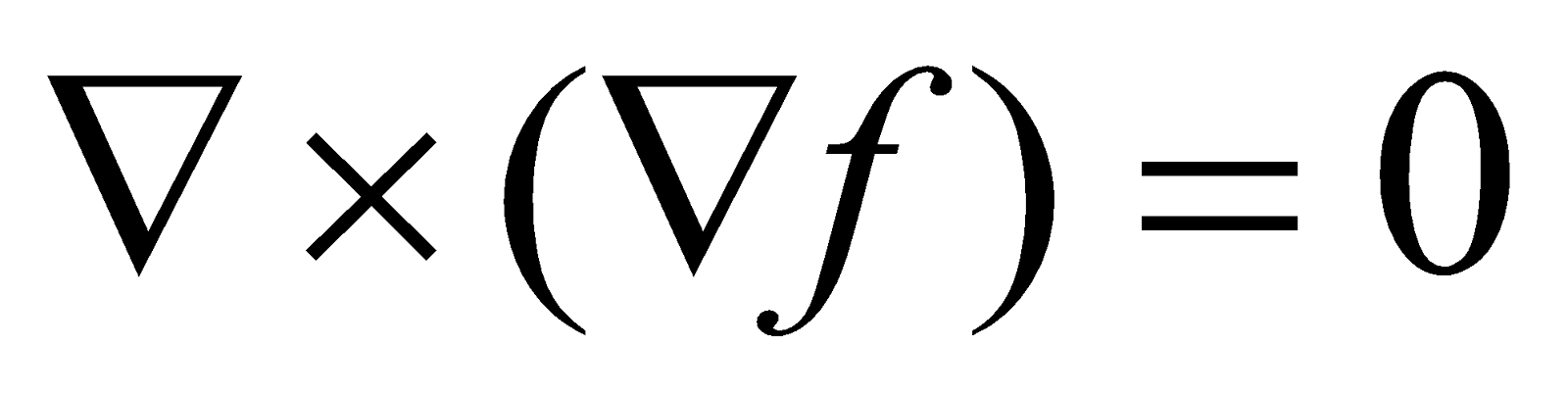## Saturday, January 05, 20192013
(November)
MATHEMATICS
(General)
Course: 101
[(a) Classical Algebra, (b) Trigonometry, (c) Vector Calculus]
Full Marks: 80
Pass Marks: 32
Time: 3 hours
The figures in the margin indicate full marks for the question
GROUP – A
(Classical Algebra)

1. Answer the following questions: 1x3=3
1. Define real sequence.
2. State the condition for convergence of infinite geometric series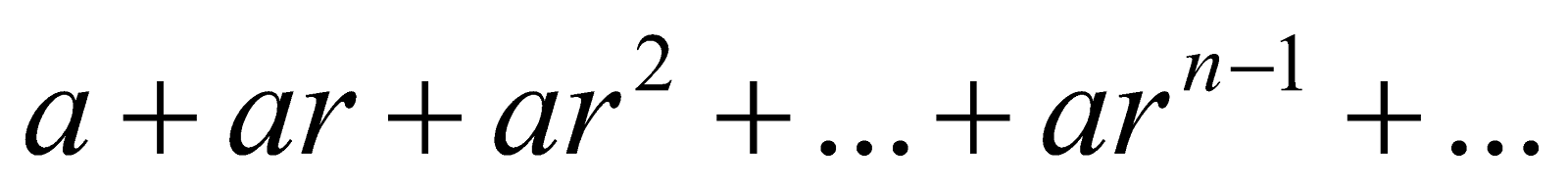1. If a polynomial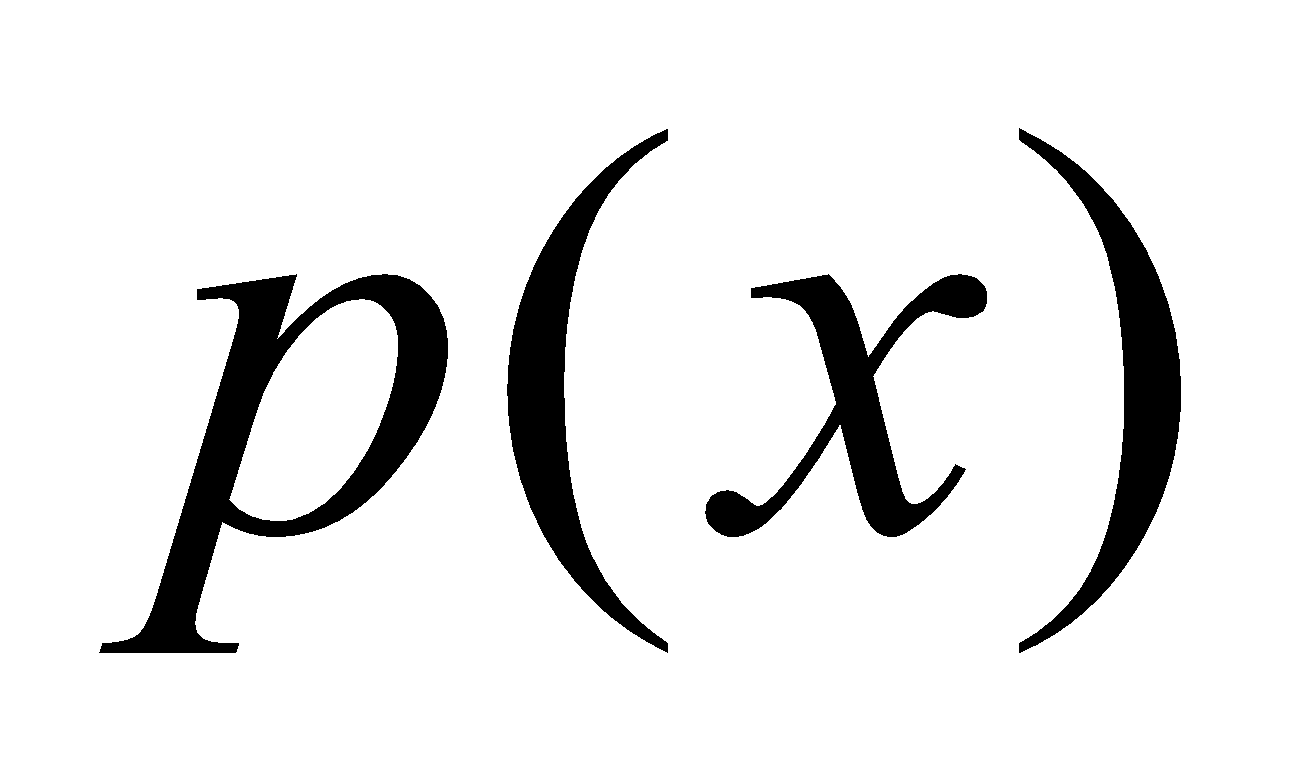is divided by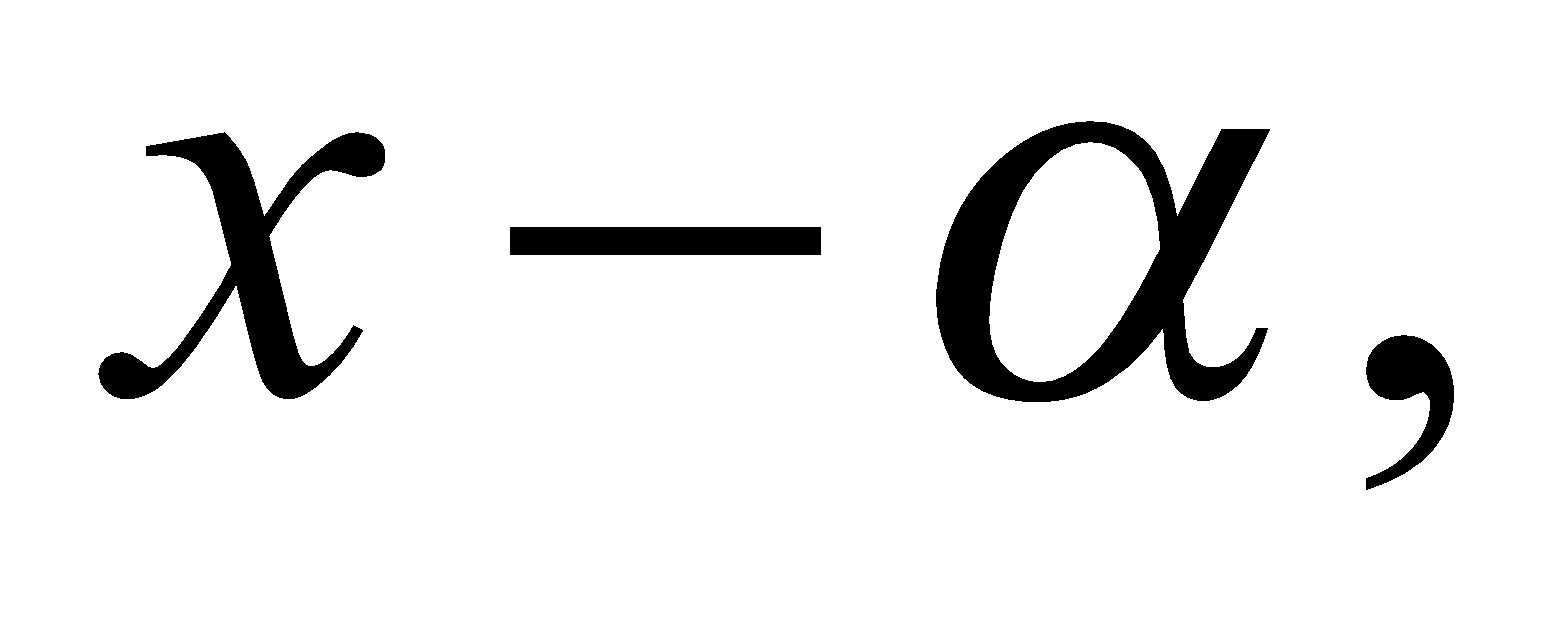where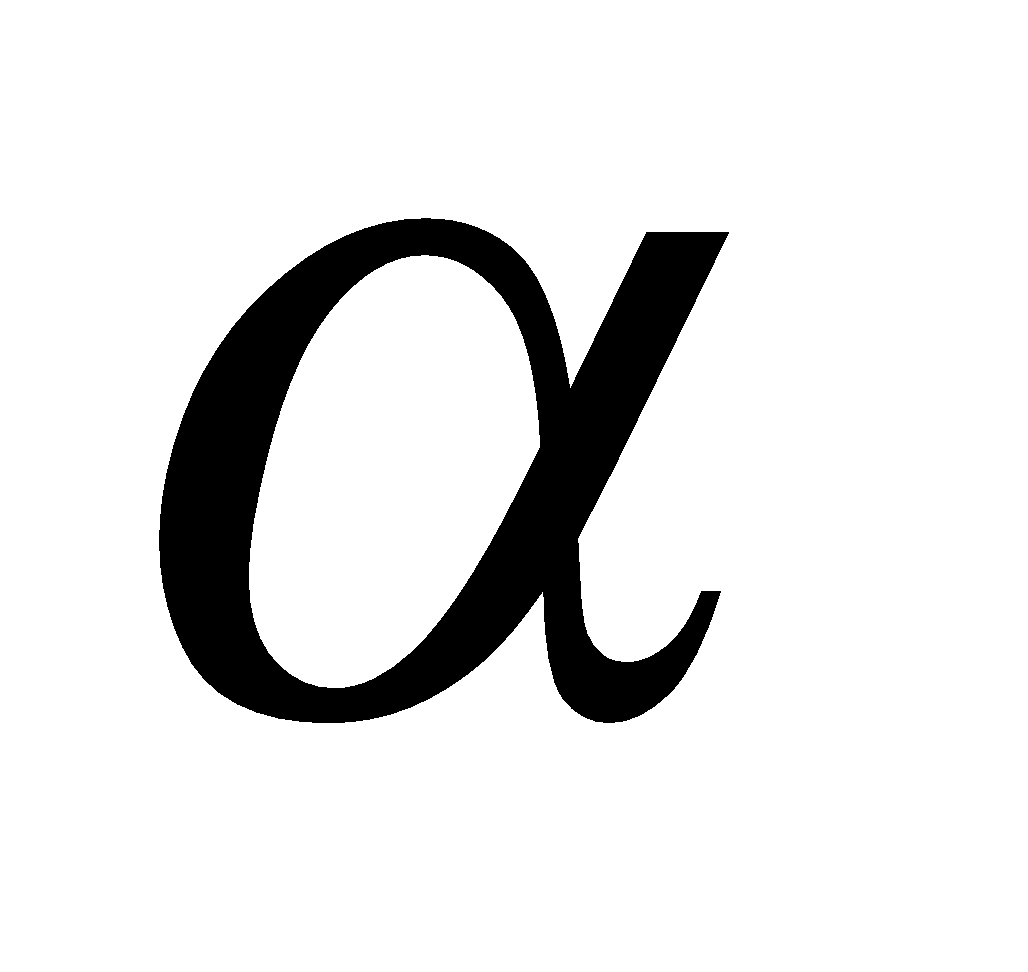is a constant, then state the remainder.
2. Answer the following questions: 2x5=10
1. Prove that a convergent sequence cannot tend to two distinct limits.
2. State the conditions of d’ Alembert’s ratio test for convergence of a series.
3. Test for convergence of the series: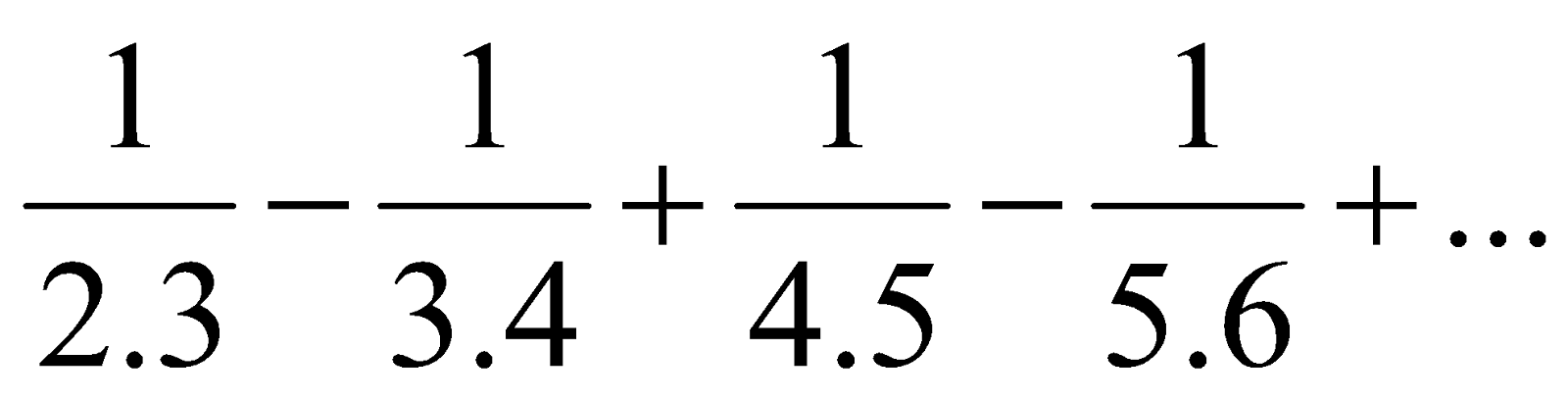1. If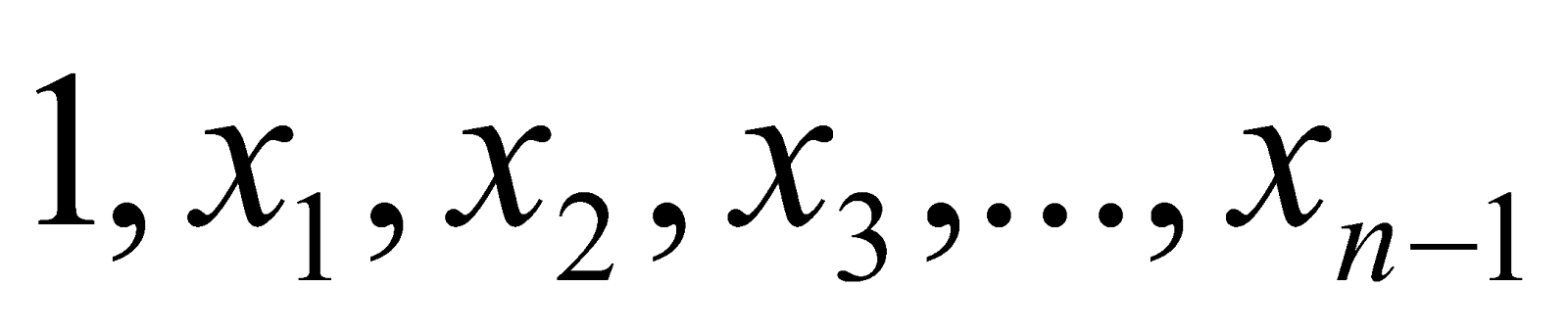are the roots of the equation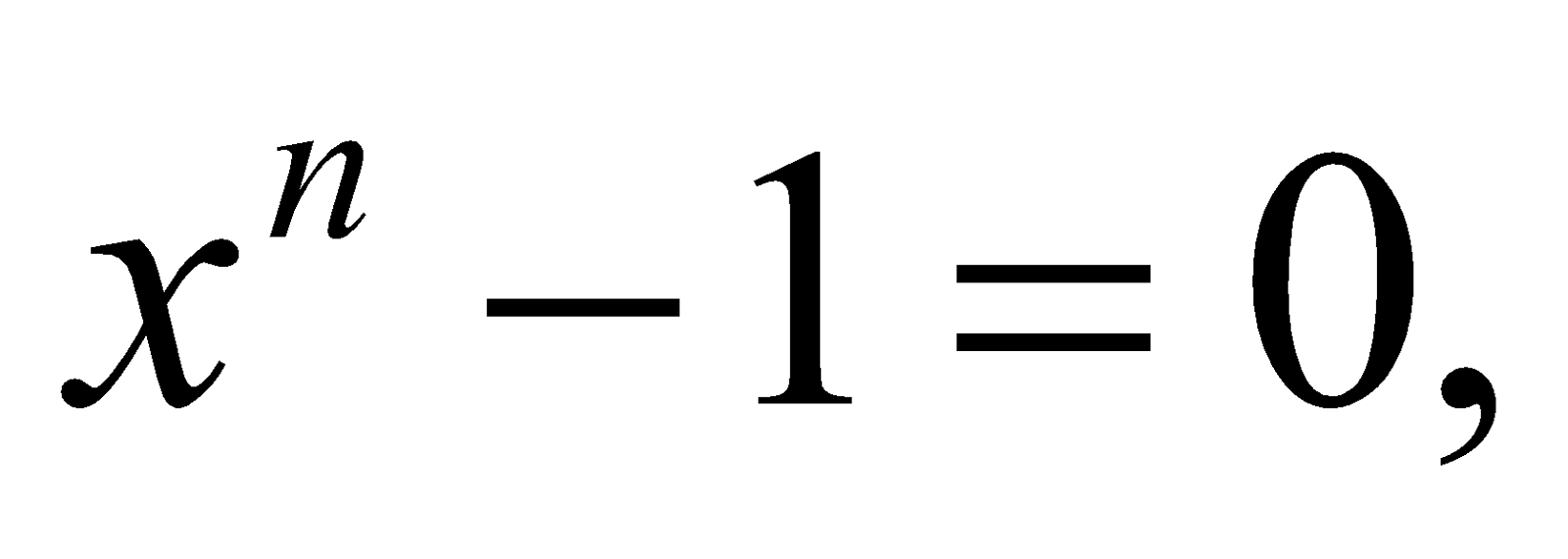then show that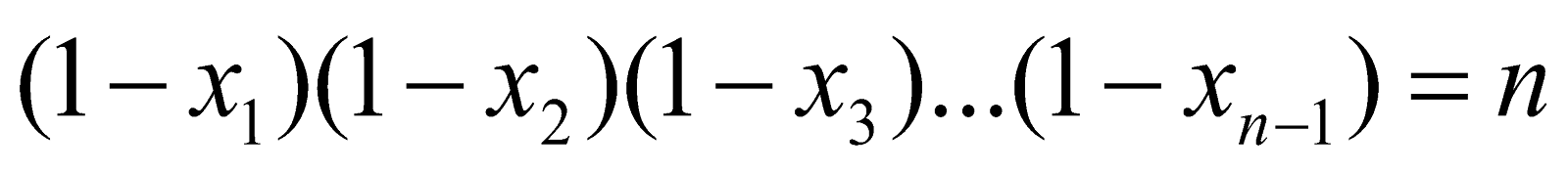1. Show that the equationhas a root between – 3 and – 2.
3. (a) Show that the sequence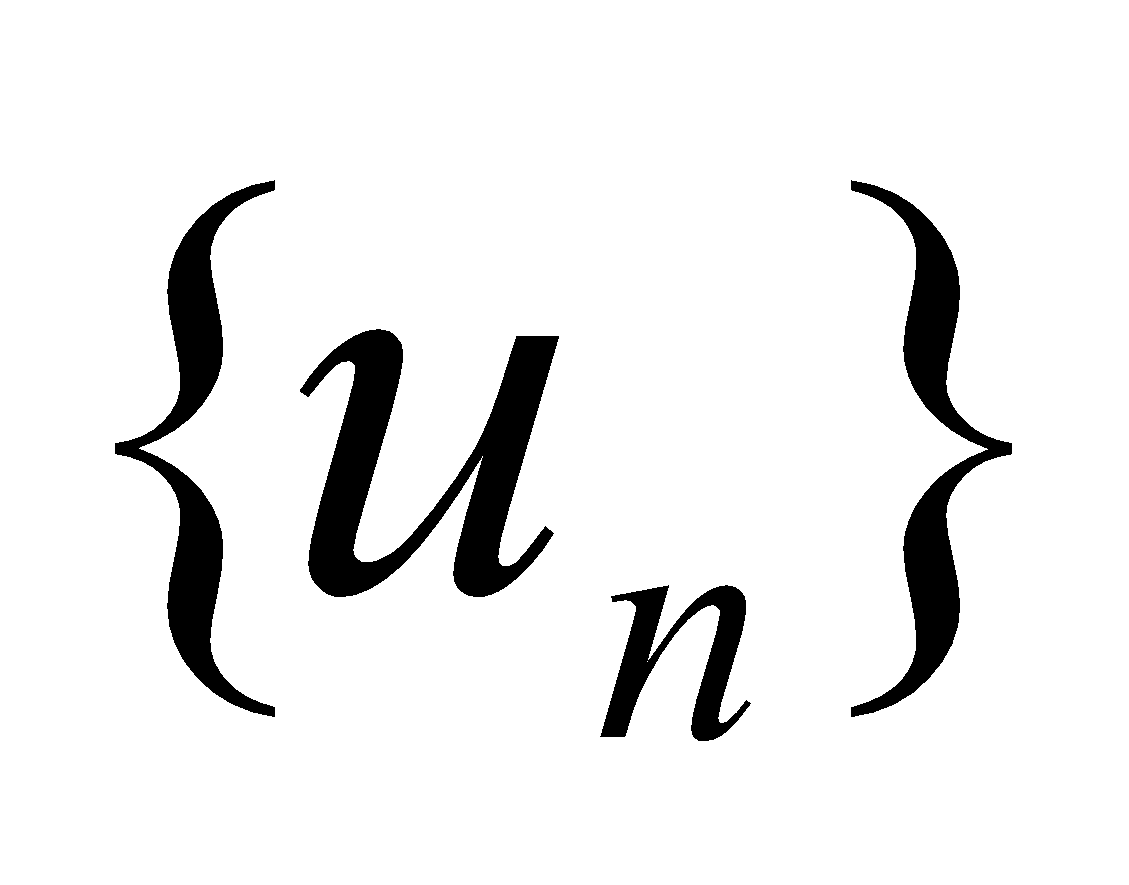is convergent, if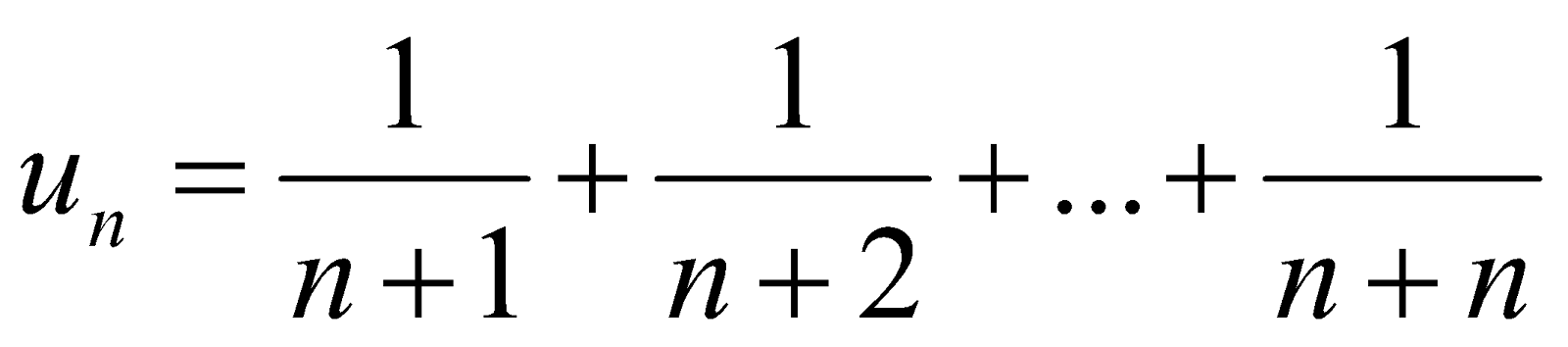3
(b) Use general principle of Cauchy’s criterion to show thatis not convergent, where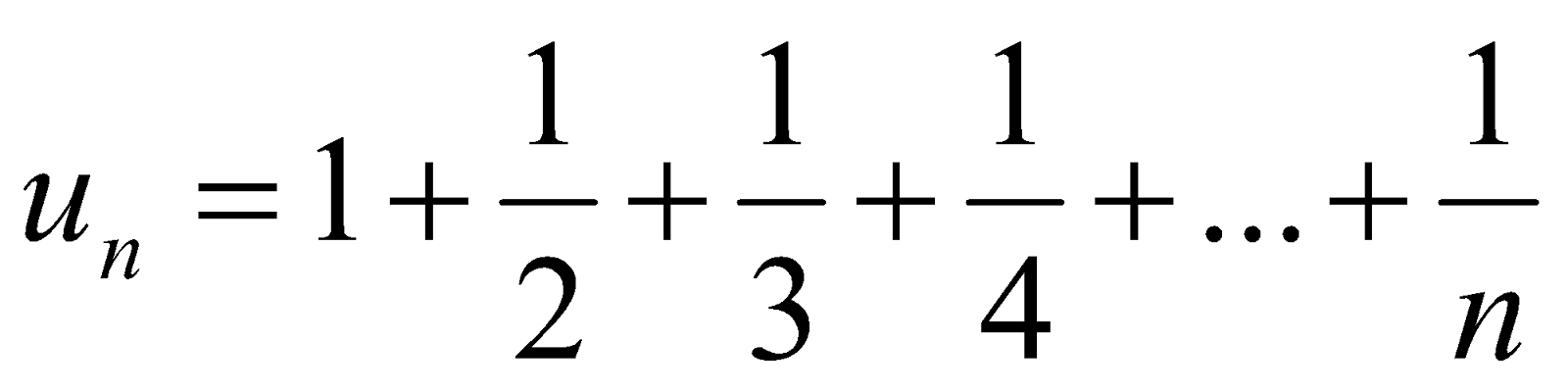4
4. Test the convergence of any two of the following: 5x2=10
1.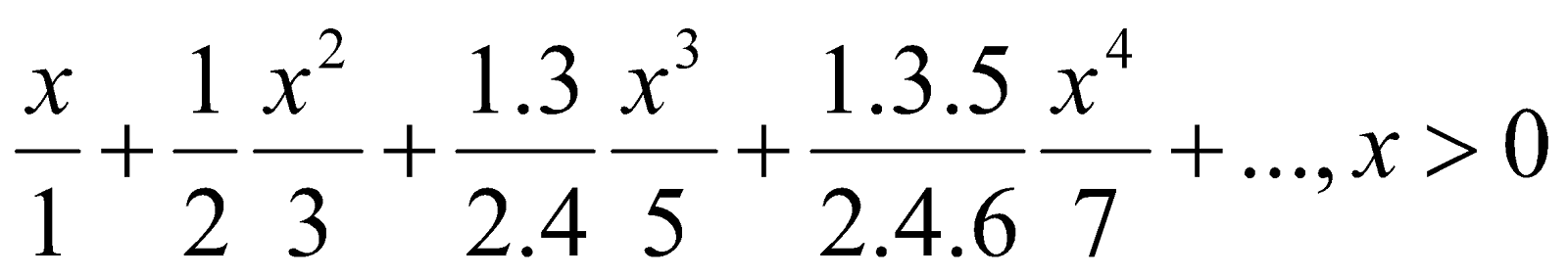2.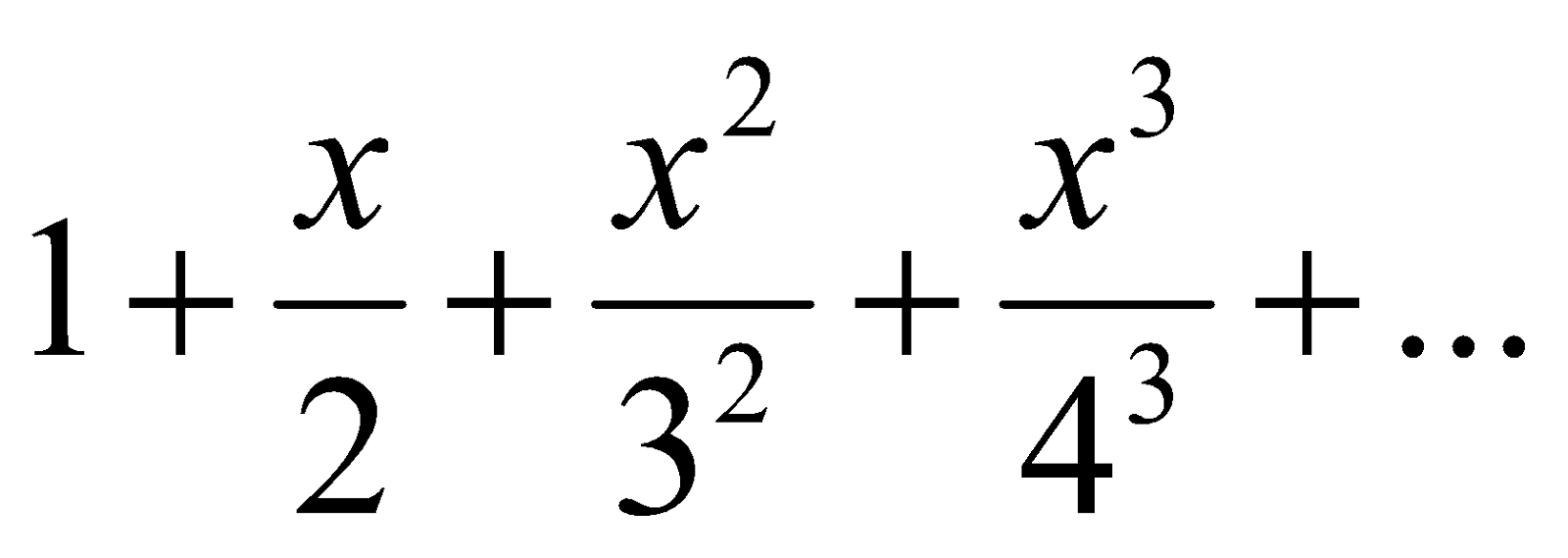3.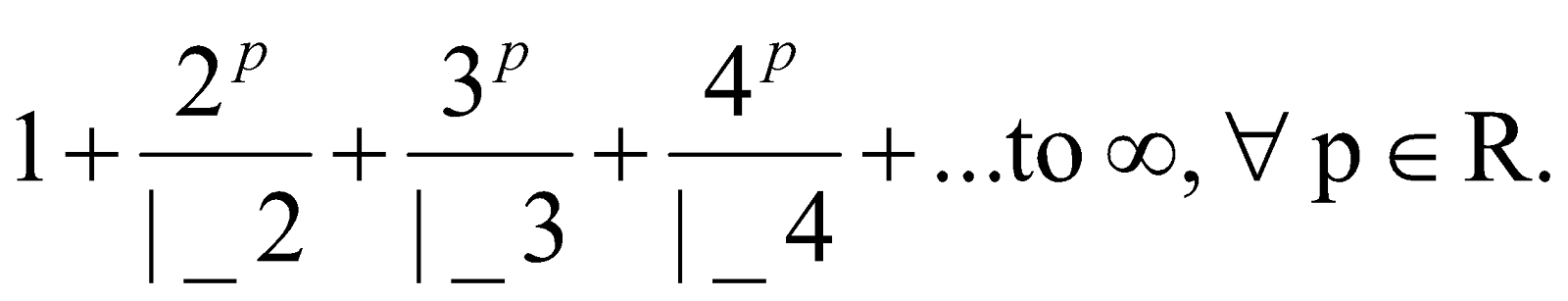5. (a) If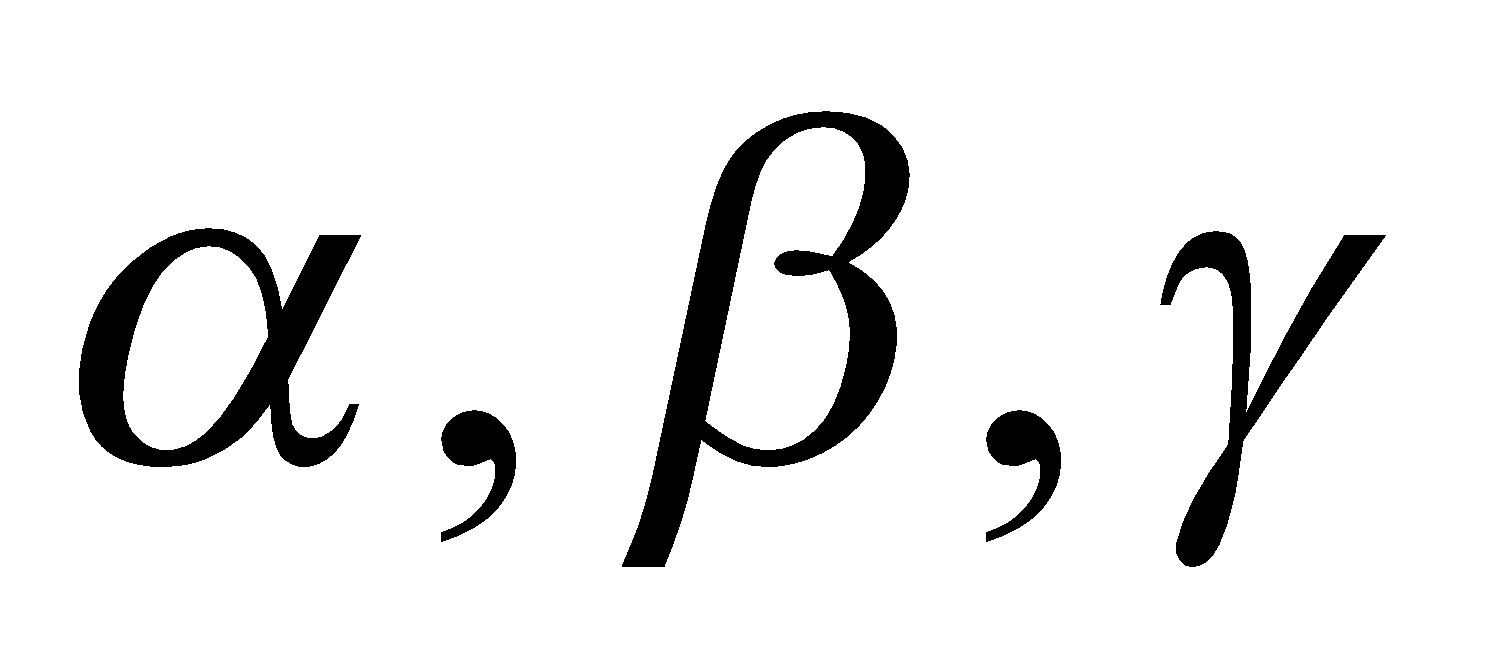be the roots of the equation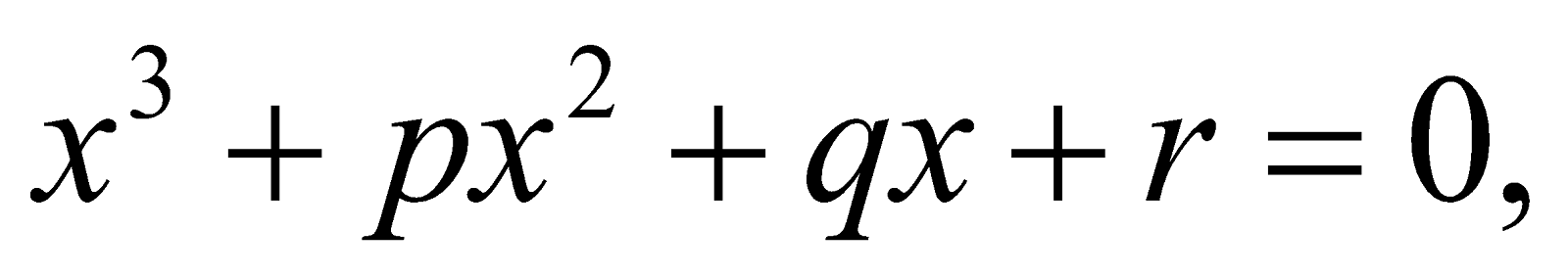form the equation whose roots are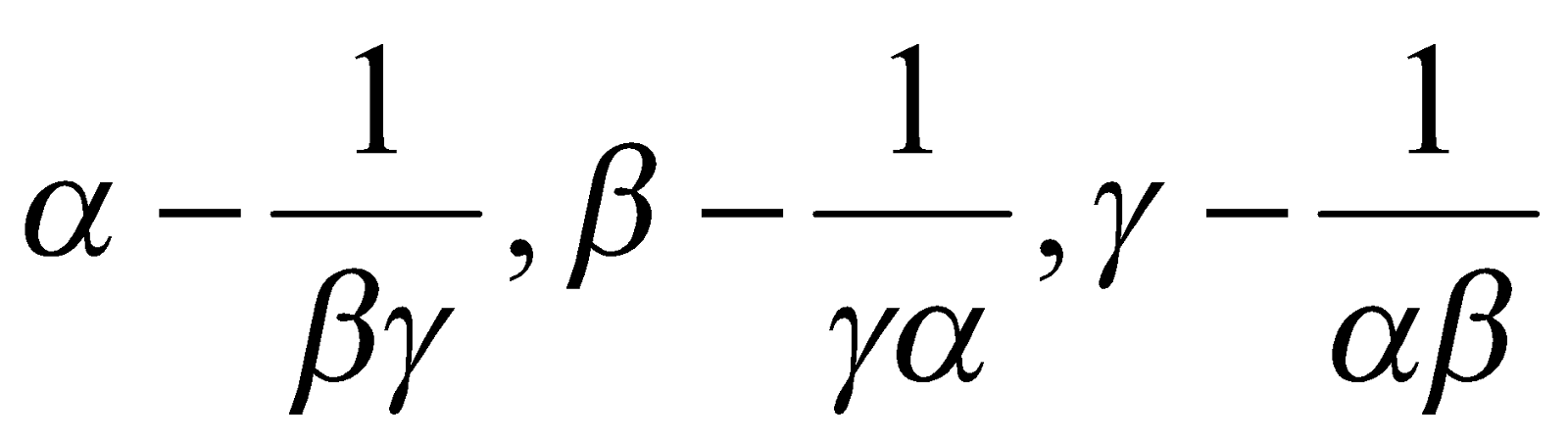.
Or
Prove that every algebraic equation of degree n has exactly n real or imaginary roots.
(b) Solve the equation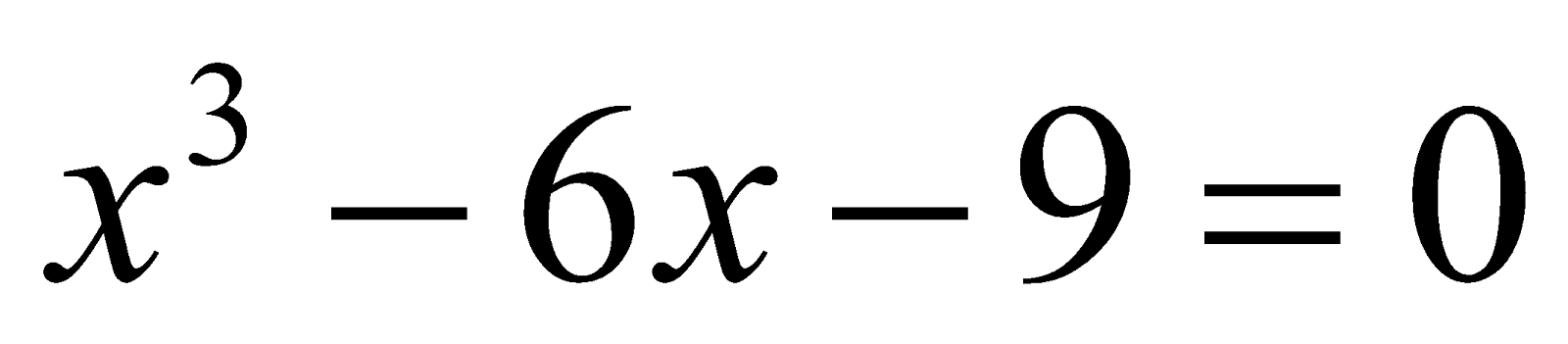using Cardan’s method.

GROUP – B
(Trigonometry)

6. (a) What is the 2nd term in the expansion of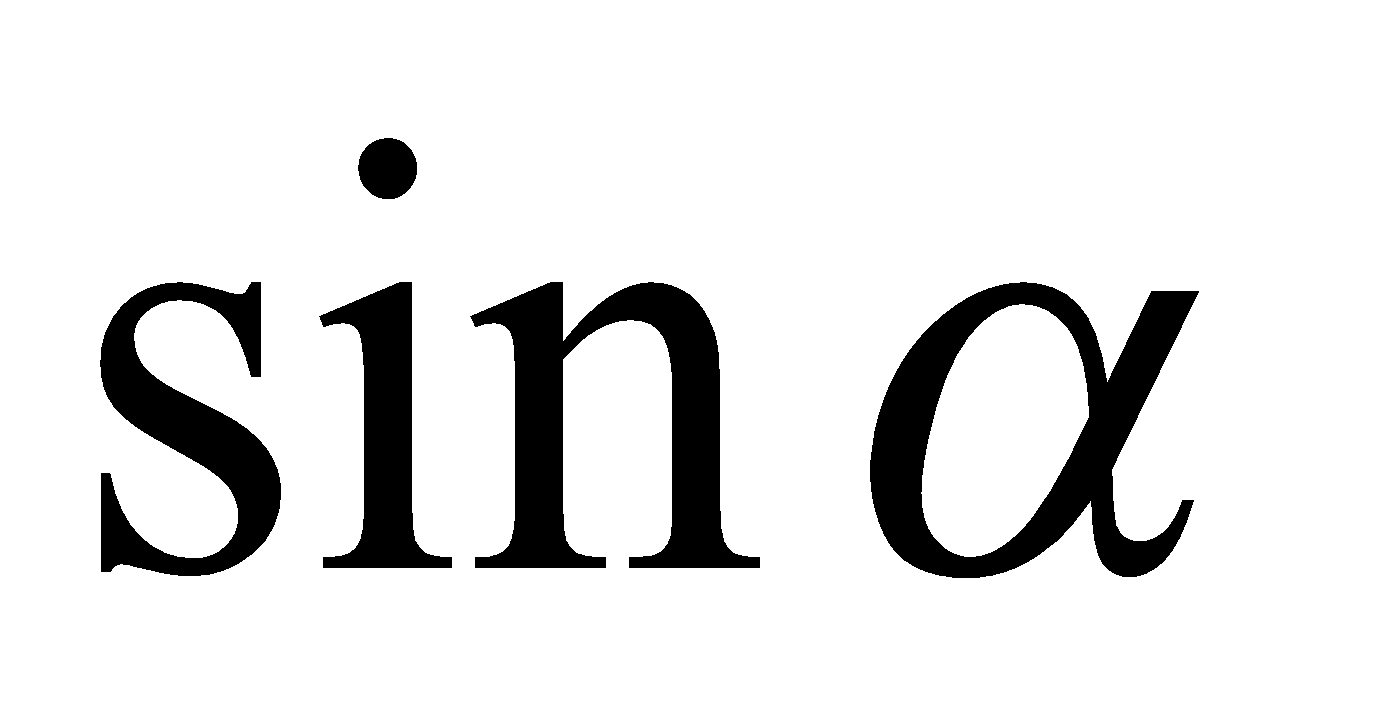in terms of?
(b) State Gregory’s series. 1
(c) Prove that 2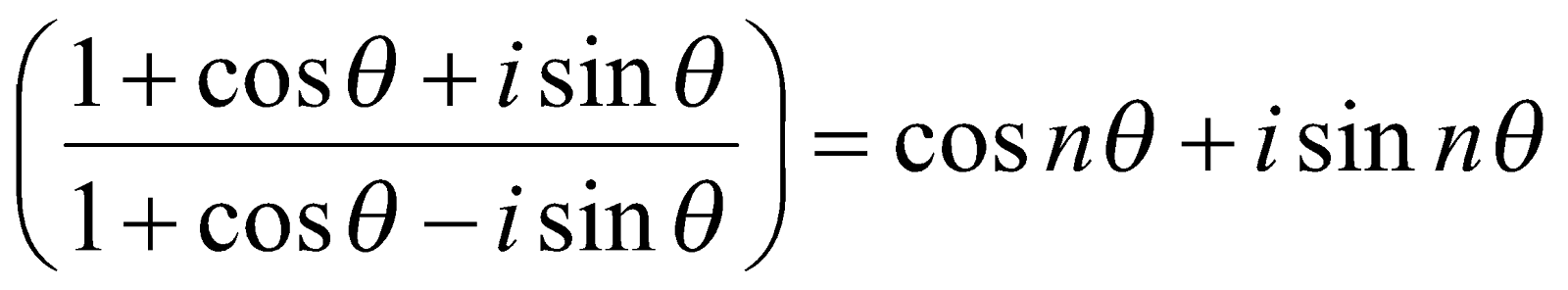7. State De Moivre’s theorem and prove it when n is any integer. 5
Or
If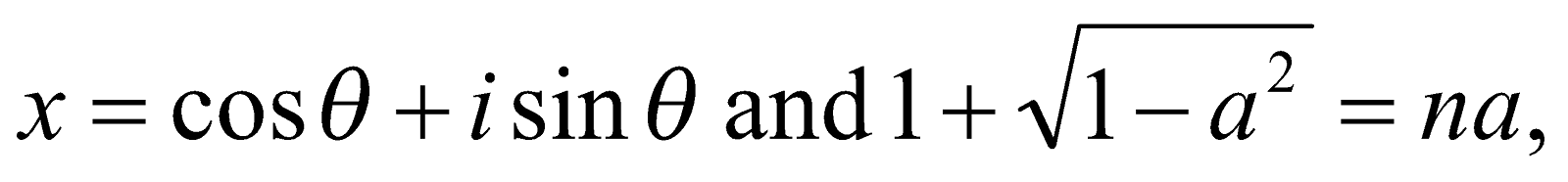Prove that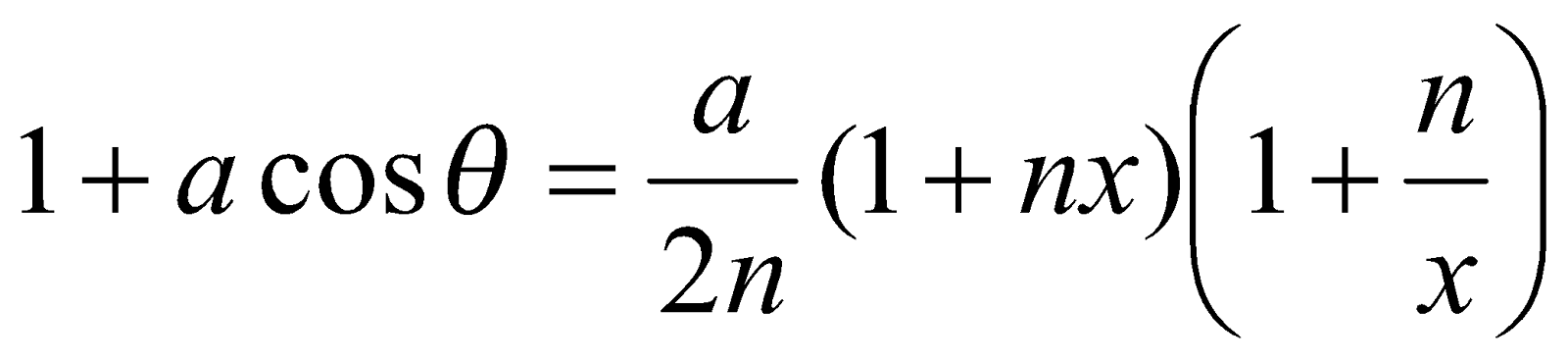8. (a) Express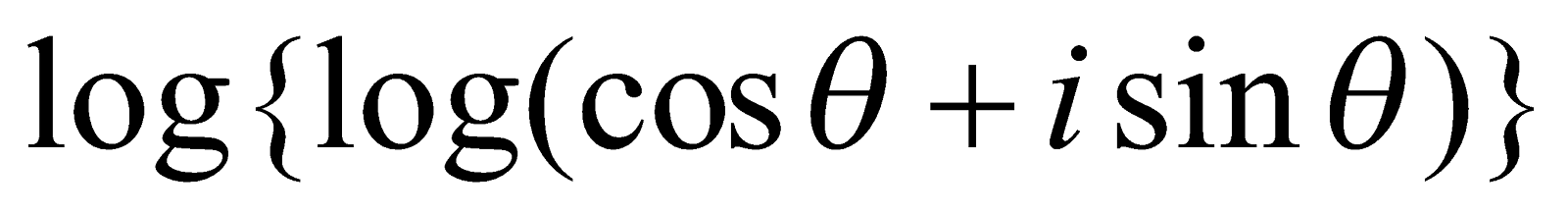in the form2
(b) Prove that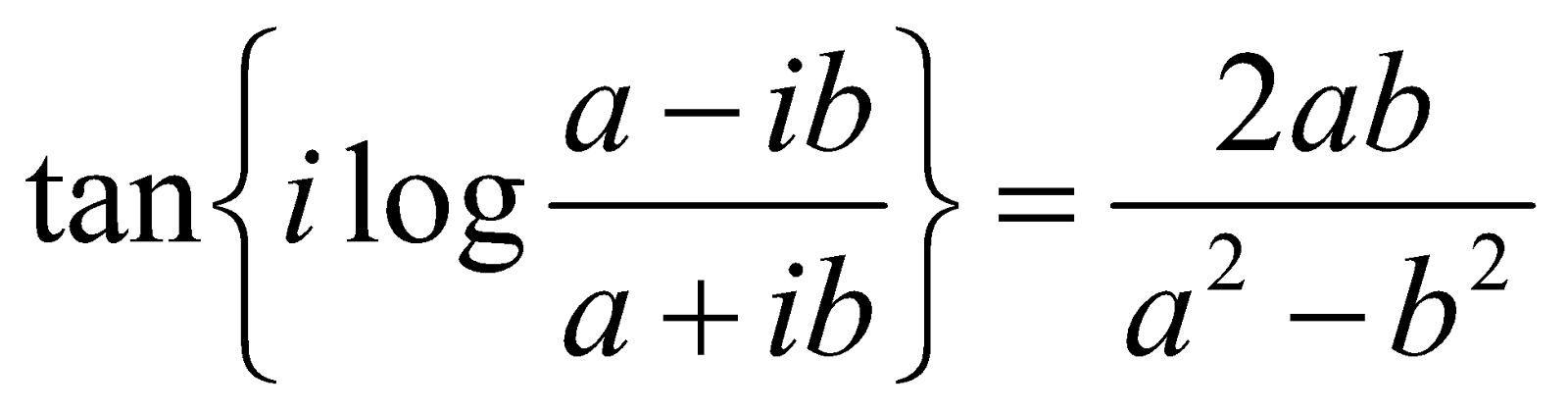where a and b are two real quantities. 3

(c) Prove that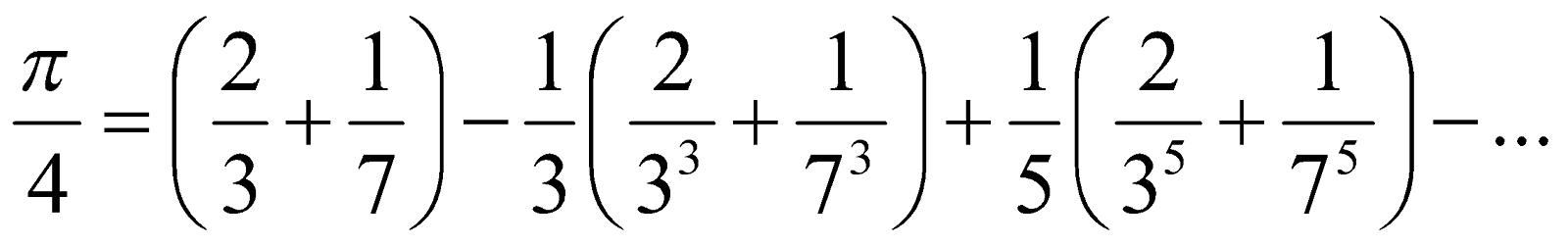3
Or
If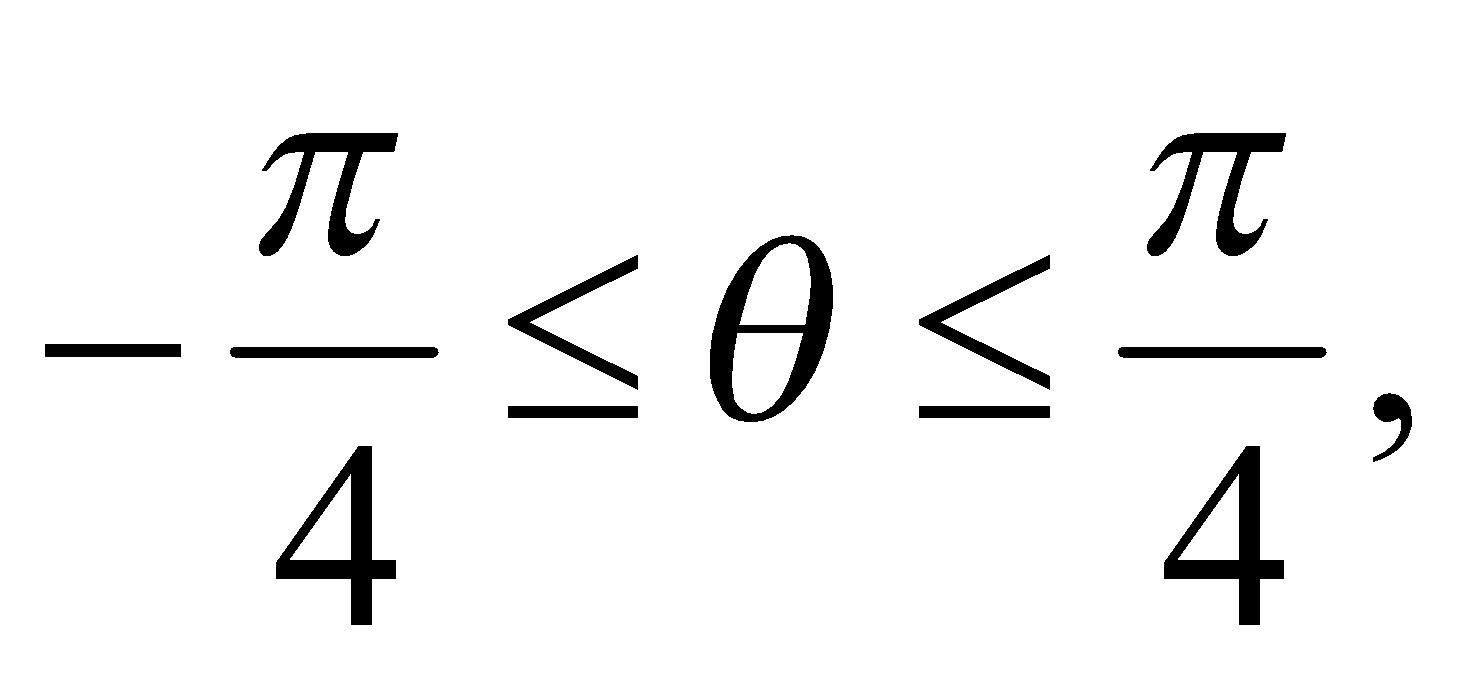prove that9. (a) If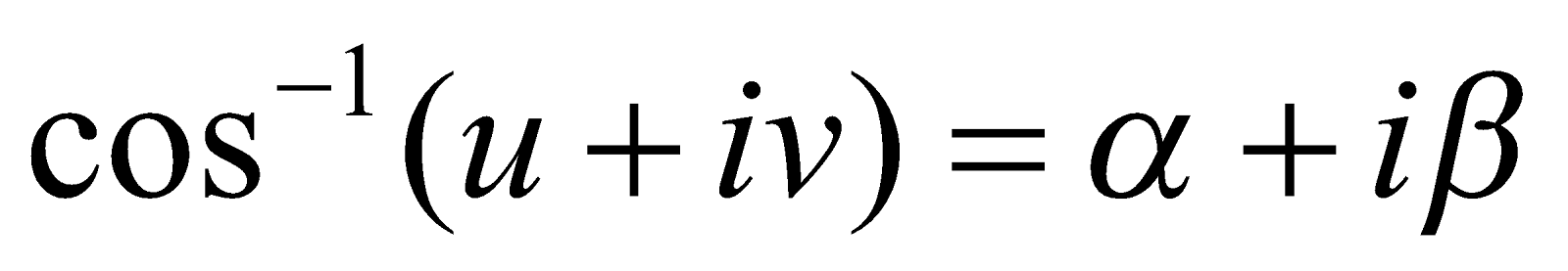, prove that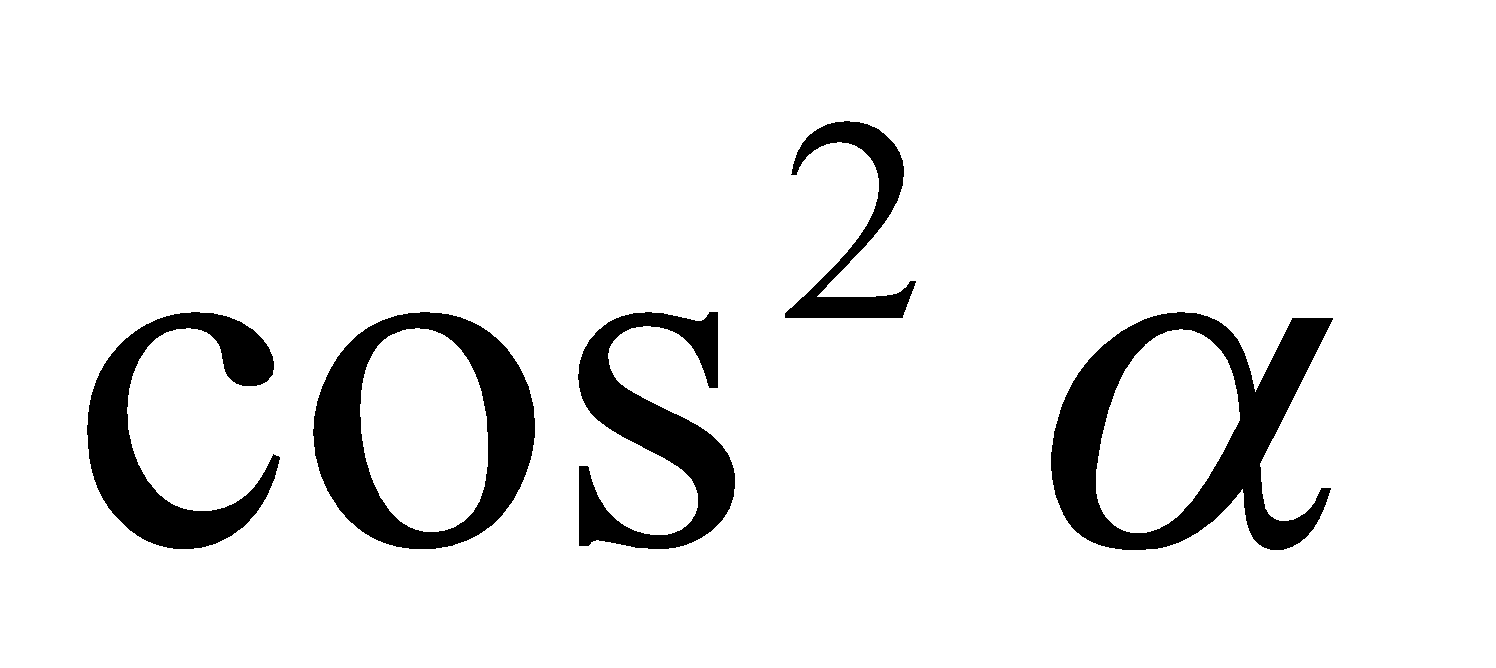and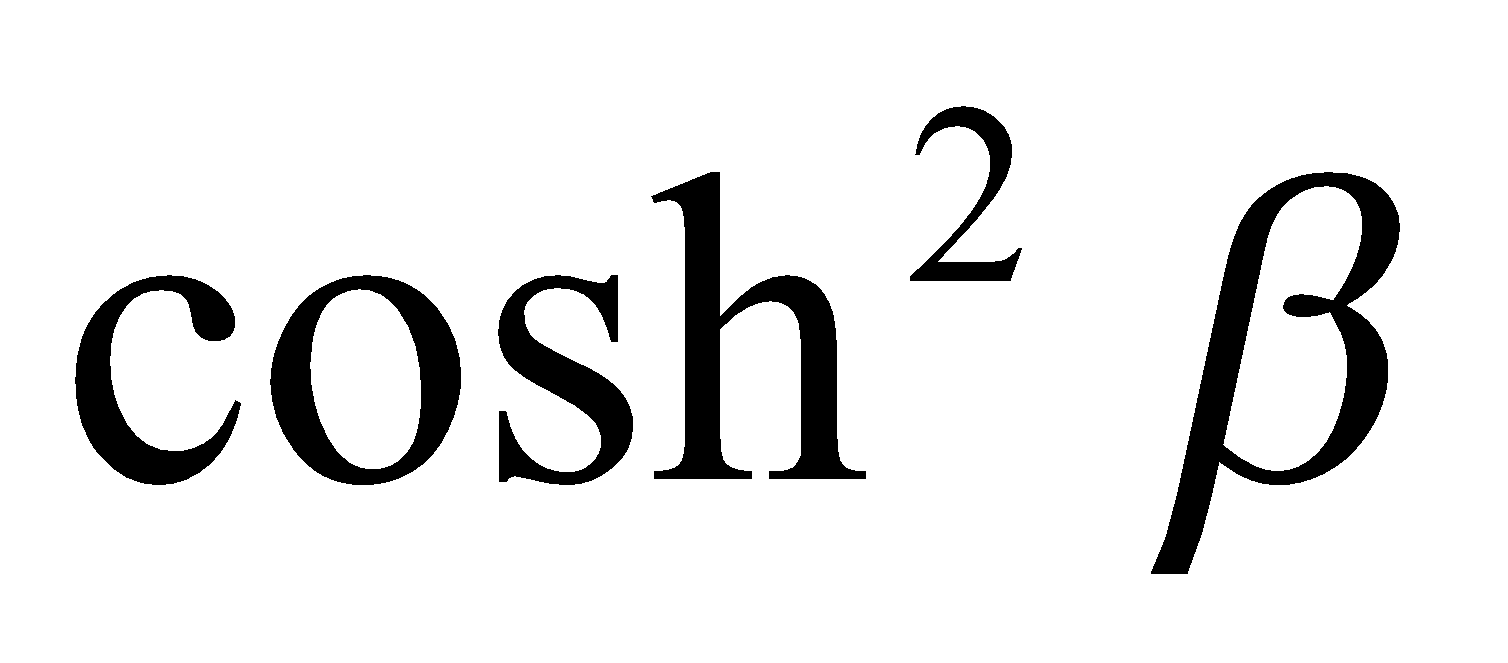are the roots of the equation 3(b) Find the sum: 5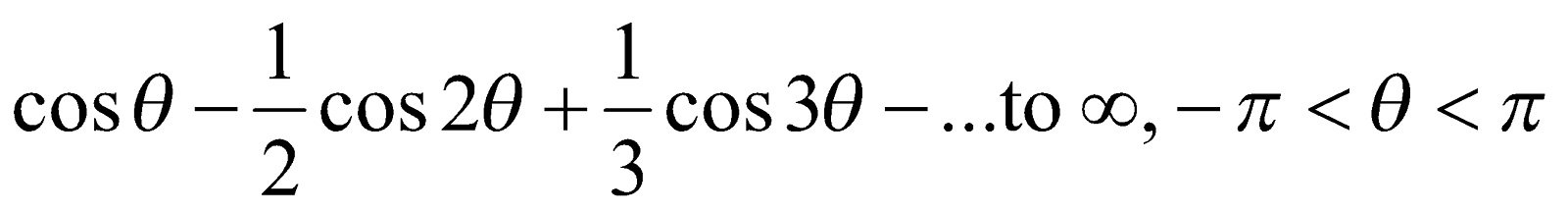Or
Find the sum of the sines of a series of n angles which are in arithmetical progression.
GROUP – C
(Vector Calculus)
10. (a) Write the following expression correctly: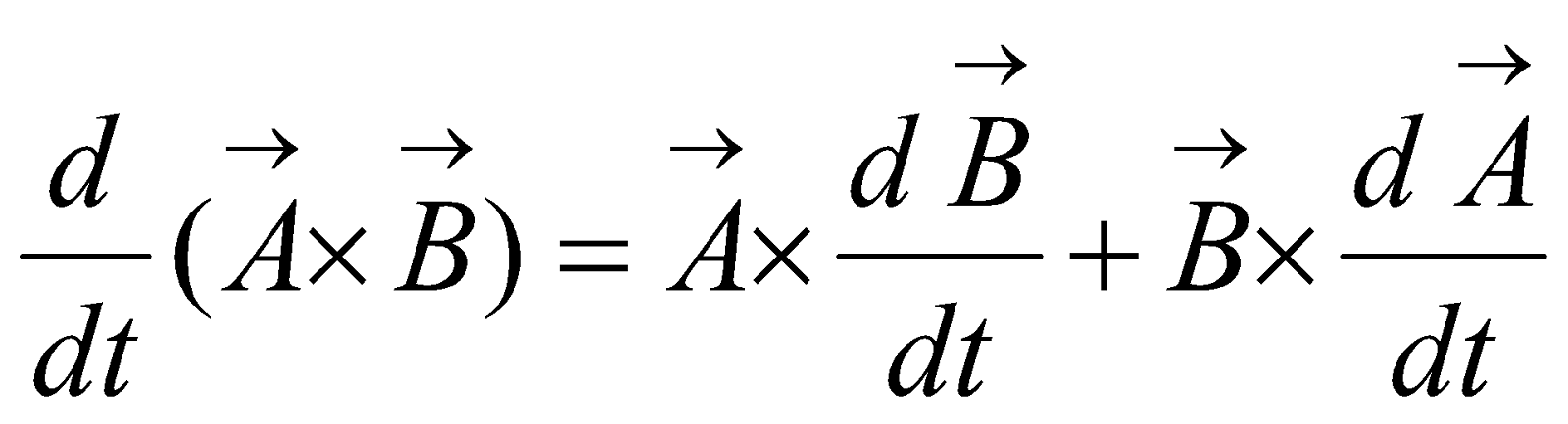1
(b) Give the definition of vector differential operator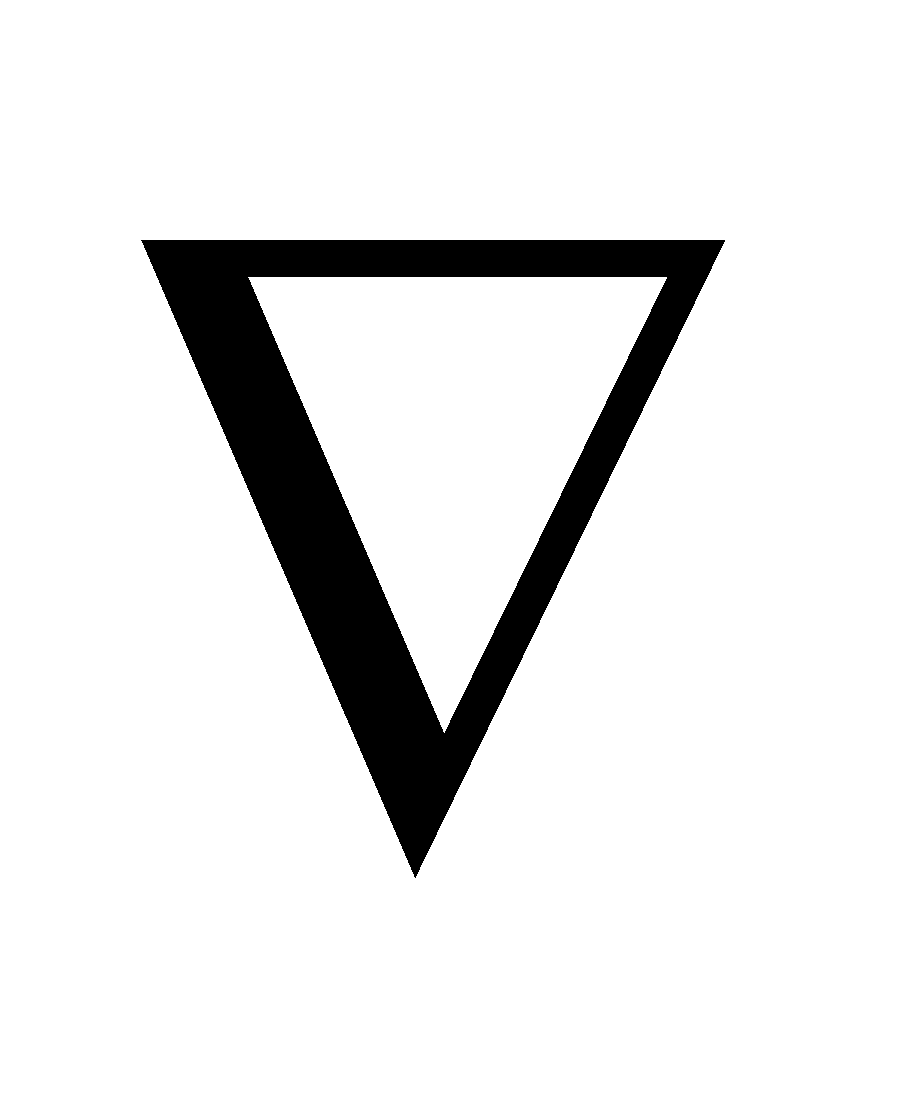. 1
(c) If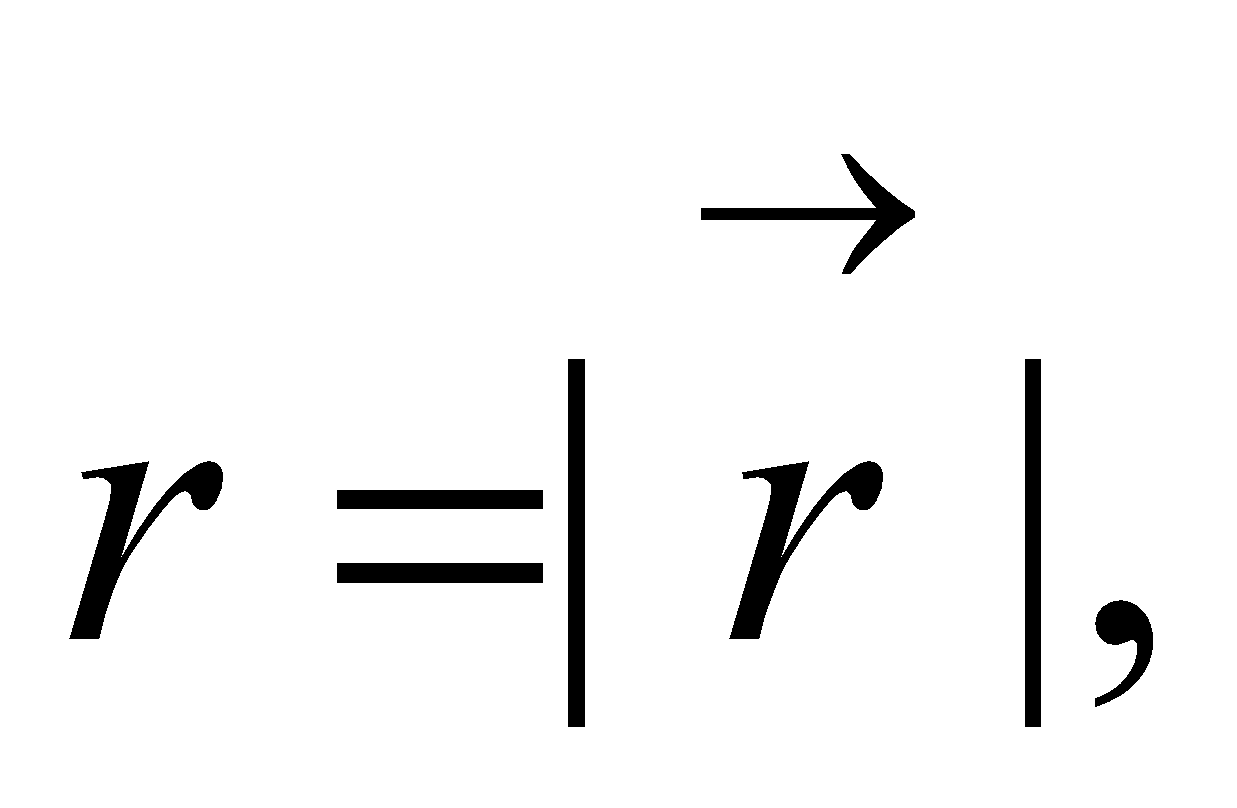where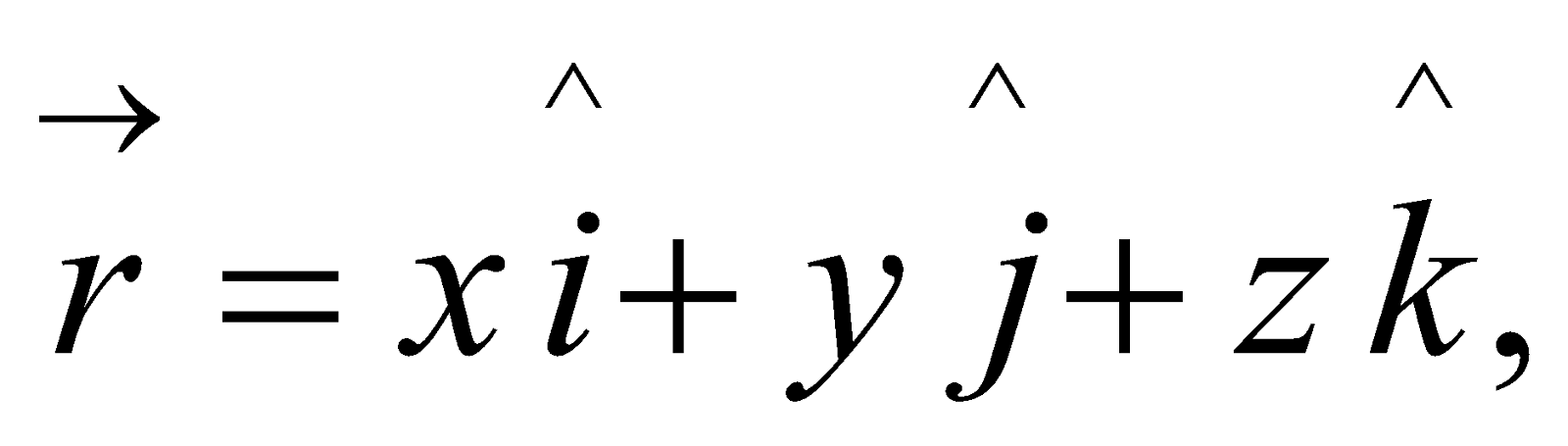prove that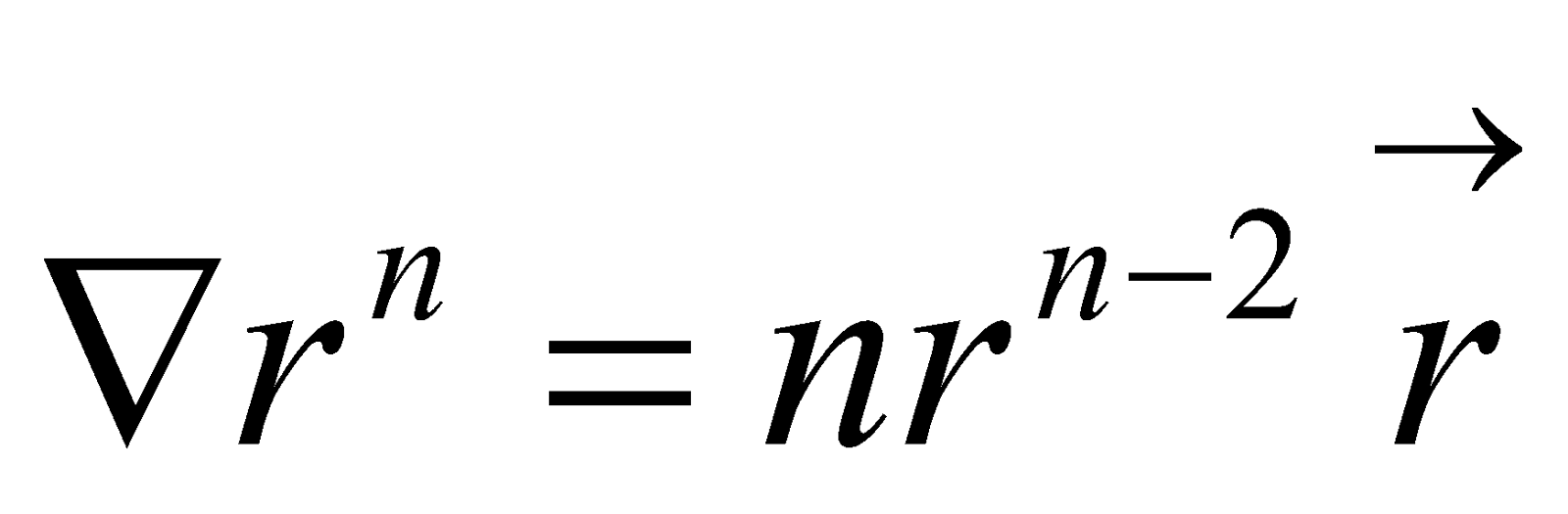2
11. What do you mean by directional derivative of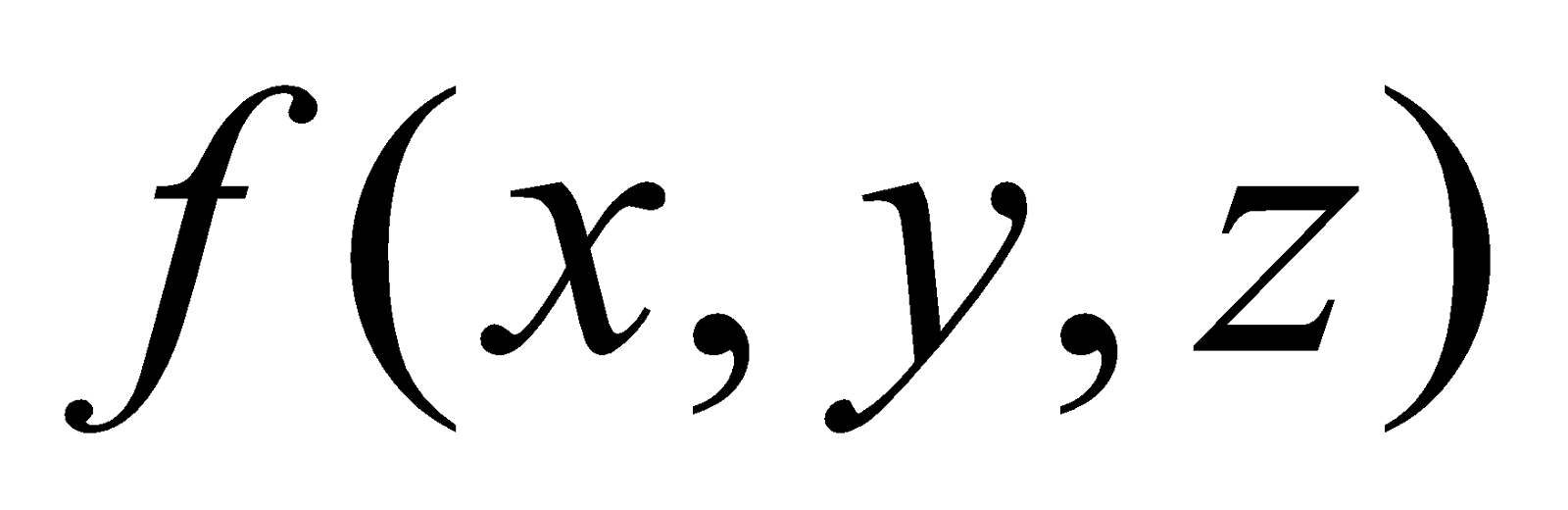at the point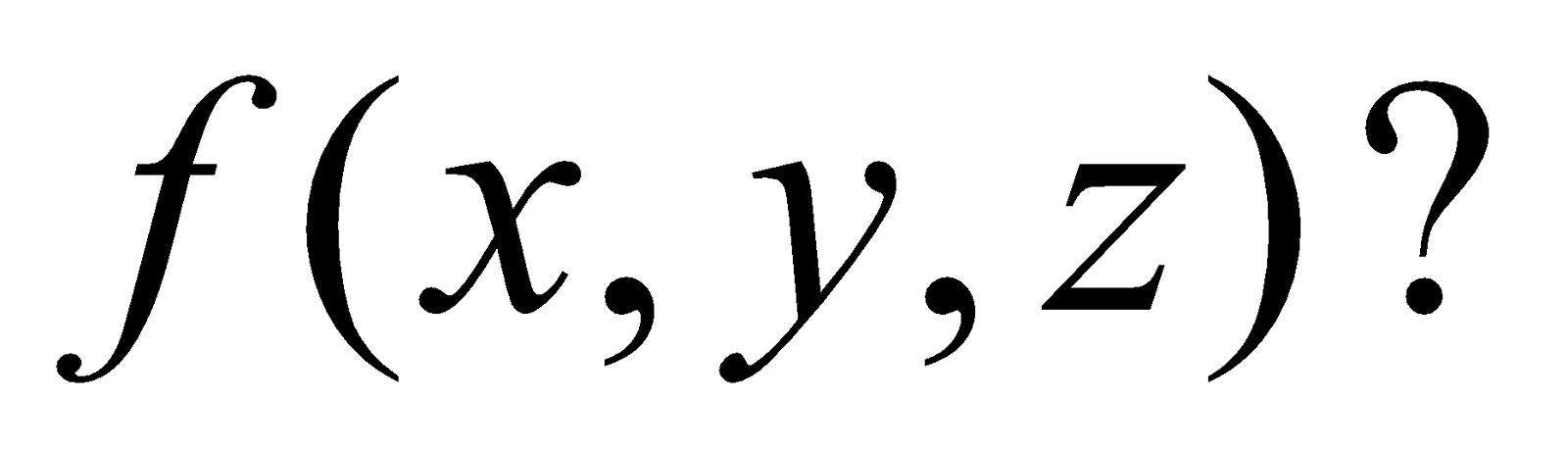Find its values in the direction of coordinate axes.
Or
Find the directional derivative of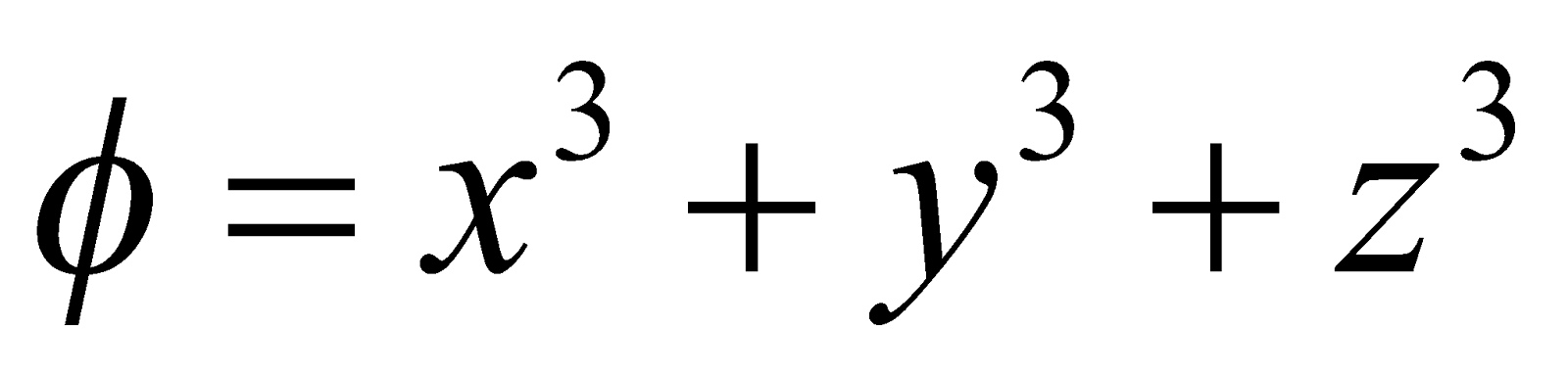at the point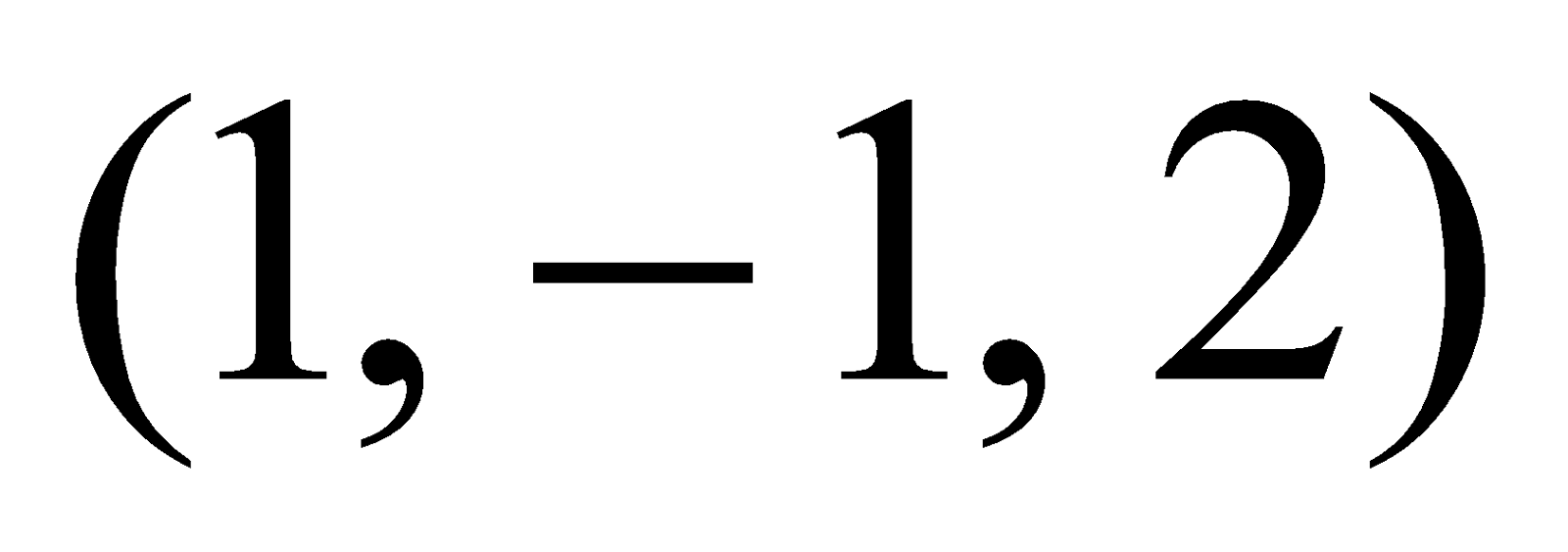in the direction of the vector12. Answer any two of the following: 4x2=8
1. Prove that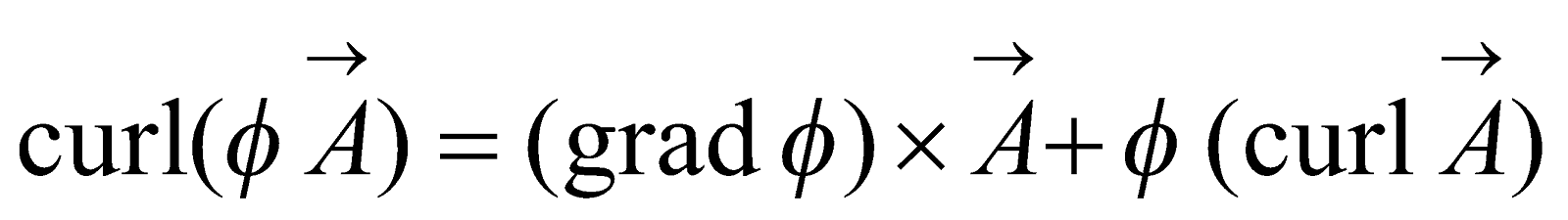2. If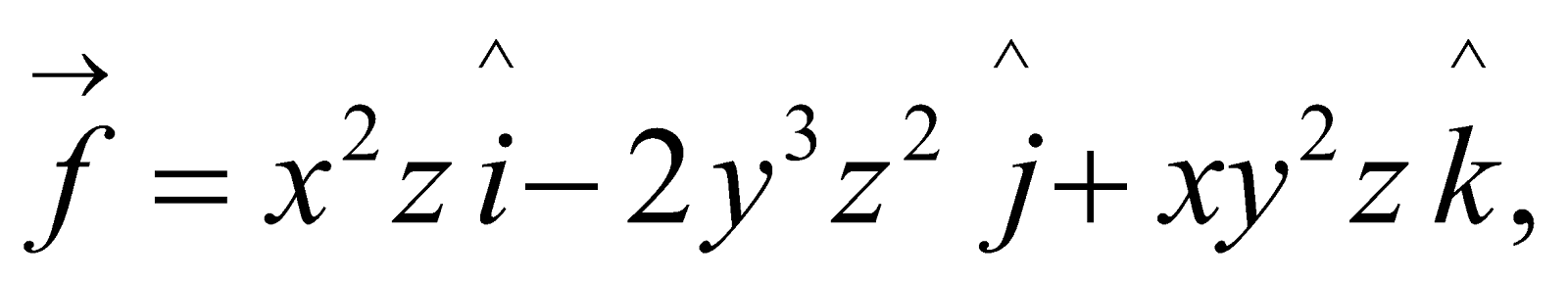determine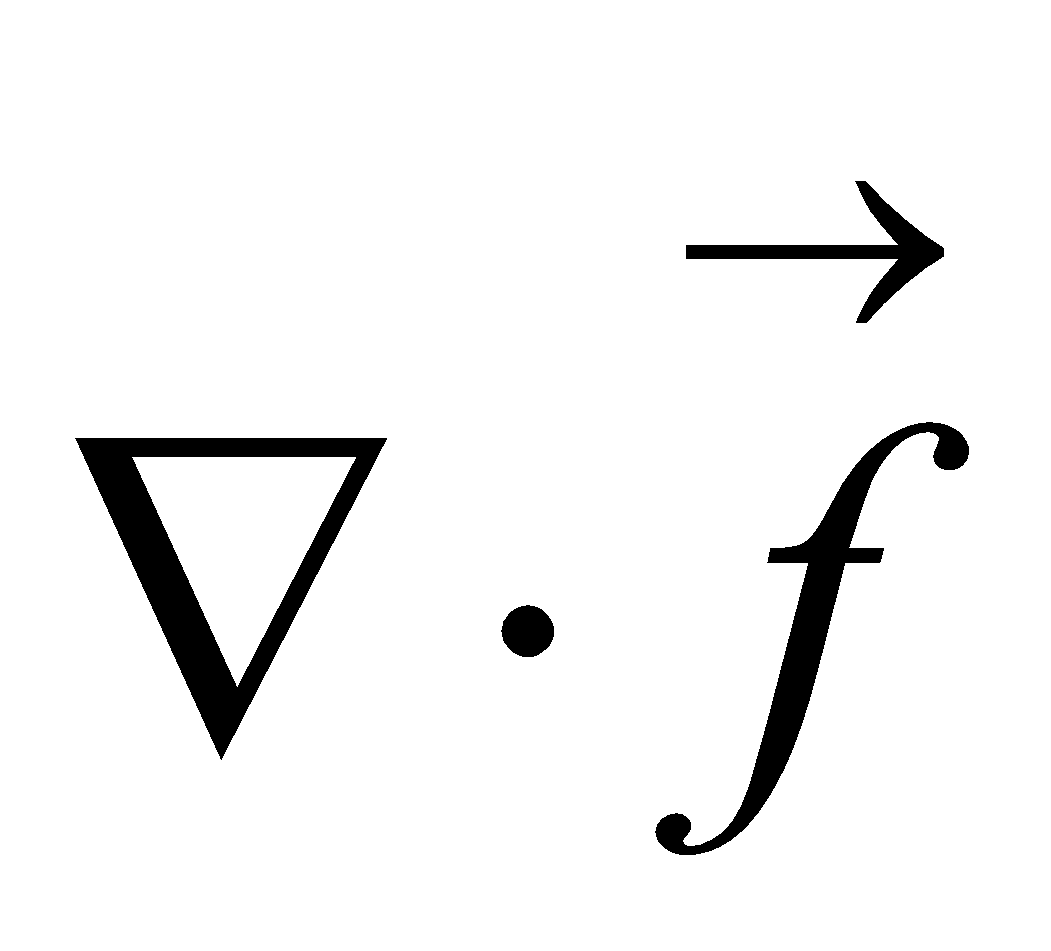and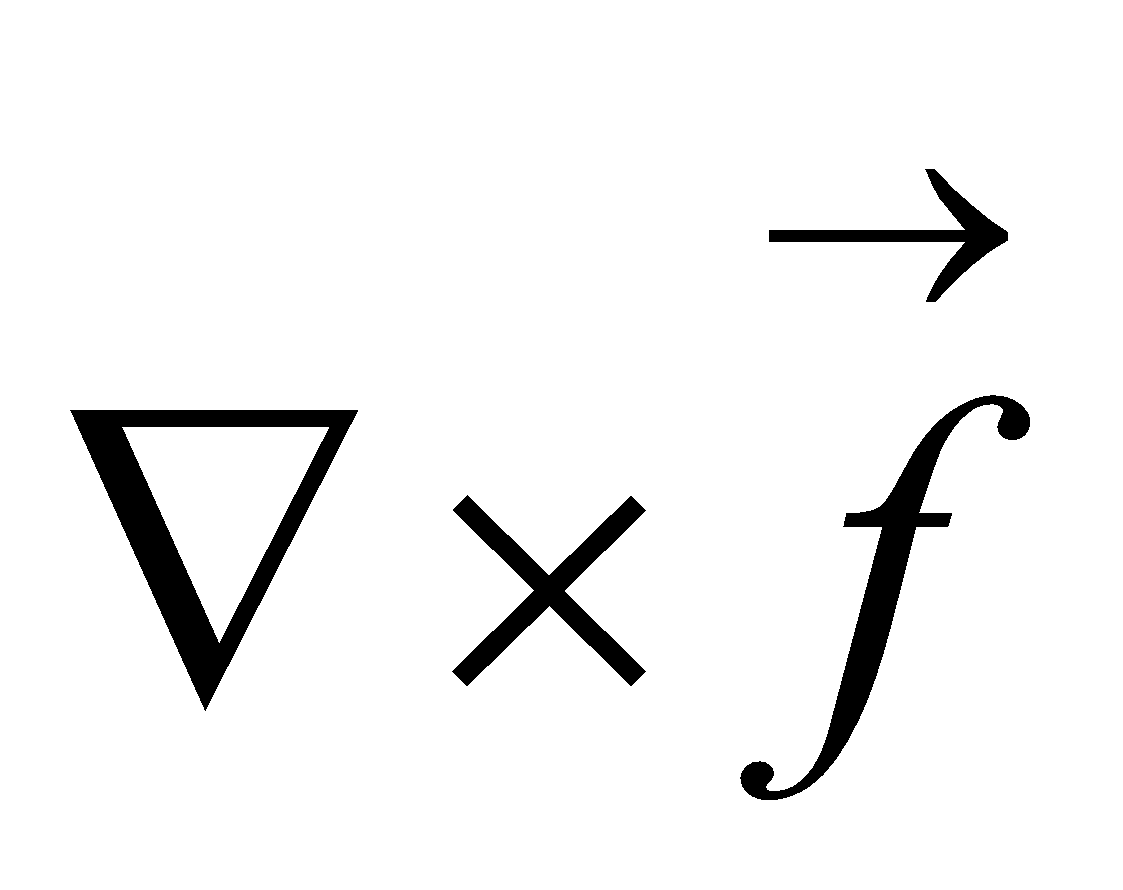at.
3. Prove that: